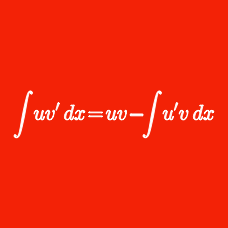Calculus

# Integration by Parts

Find the indefinite integral $\displaystyle{ 43\int{x \ln x dx} },$ using $C$ as the constant of integration.

Evaluate the integral $\displaystyle{ 128\int{x \cos 3x dx} },$ using $C$ as the constant of integration.

Find the indefinite integral $\displaystyle{ \int{x \sqrt{x+19} dx} },$ using $C$ as the constant of integration.

Evaluate the integral $\displaystyle{ 67\int{t^2 e^{3t} dt} },$ using $C$ as the constant of integration.

Evaluate the integral $\displaystyle{ 43\int _{ 0 }^{ 1 }{ \arctan x dx } },$ using $C$ as the constant of integration.

×